# What is the density of the liquid

If you compare cubes made of different metals, all of which have a volume of exactly one cubic centimeter, you can see that the cubes have different masses. The aluminum cube is significantly lighter than the copper, iron or zinc cubes.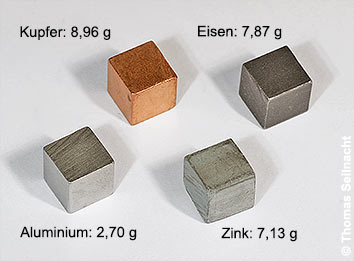Different metal cubes with the same volume

One liter of water has a mass of one kilogram (kg), while one liter (l) of ethanol weighs only about 789 grams (g). The alcohol has a lower density than the water. The density is calculated from the quotient of the mass of a body and its volume:

Density = mass ÷ volume
SI standard: ρ = kg ÷ m3
Common: ρ = g ÷ cm3 or ρ = kg ÷ dm3

The abbreviation for the density is the Greek letter rhoAccording to the international SI standard, its unit is kilograms per cubic meter (kg / m3). The unit used in chemistry books is grams per cubic centimeter (g / cm3) or kilograms per cubic decimeter (kg / dm3). Depending on the unit, the value at +4 ° C for water is ρ = 1000 (SI standard) or ρ = 1 (common). Like mass, density is a variable that is independent of location. Volumes depend on the temperature. Therefore, the temperature is always given for the density. The density data in the tables generally relate to +20 ° C. By rearranging the equation, each of the quantities can be calculated.

Density determination by weighing and volume determination

The easiest way to determine density in the laboratory is to precisely determine the mass and volume of a body and then calculate it using the above equation. The volume of gases and liquids is determined with the usual methods for volume determination. In the case of solids, the volume can be calculated based on the special shape, for example in the case of cubes, cuboids or slices. Another possibility is the immersion of bodies in measuring cylinders filled with water. The increase in the liquid level shows the actual volume of the body.

Density determination with the hydrostatic balance

According to the Archimedes' principle, a body immersed in a liquid generates a force directed vertically upwards. This force is called buoyancy. The size of the buoyancy corresponds to the weight of the displaced liquid. In addition, the body apparently loses as much weight as the volume of fluid it displaces.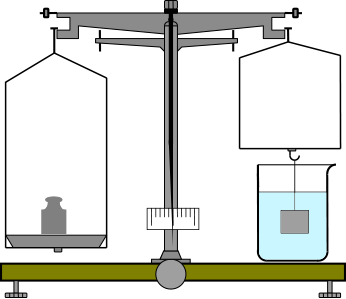Hydrostatic balance

The hydrostatic balance is used to measure the density. The mass of a body is first determined in the air and then weighed again to determine the weight of the body immersed in the water. The difference between the two weighings corresponds to the buoyancy exerted on the body and, at the same time, the weight of the displaced water. Since the density of the water is 1 g / cm³, the volume of the displaced water and thus of the body can be determined. Example:

A metal piece weighs 100 g in the air and 80 g in the water of the hydrostatic balance. The body therefore displaces 20 g of water, which corresponds to 20 cm³ of water. The volume of the metal piece is therefore 20 cm³. Now you can easily determine the density of the piece of metal.

The Mohr-Westphalsche balance is a balance that shows the density of the body directly after immersion in the water. Hydrostatic density determinations are only possible with bodies that have a higher density than water.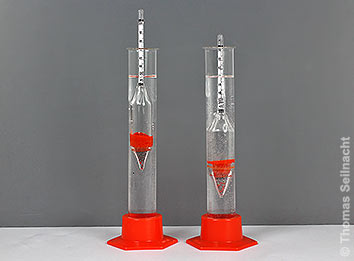Hydrometers float on two liquids.

Density determination with a hydrometer

Hydrometers are also called “spindles”. You determine the density using the floating method: If the hydrometer is placed in a liquid so that it floats, the density can be read directly from the scale using the depth of immersion. For the exact determination of the density, it is advisable to purchase a whole set of hydrometers that are calibrated to a certain temperature. The density of an alcoholic drink and thus the alcohol content can also be determined in the manner described. An alcoholometer is a hydrometer specially designed for alcohols.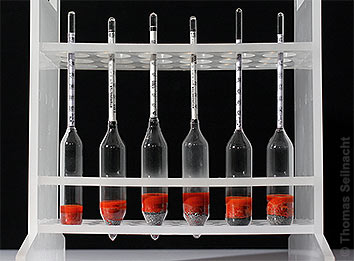Set of different hydrometers

Density determination with a pycnometer

Pycnometers are containers calibrated to a certain temperature into which a very precise volume of a certain amount of liquid can be filled. As a rule, they are calibrated at 20 ° C. A thin tube is embedded in the stopper so that the pycnometer can be filled without air bubbles.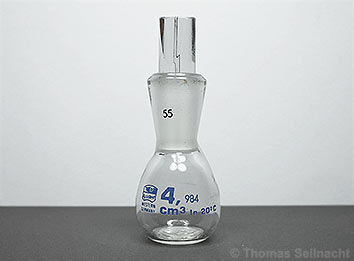Pycnometer with a volume of 4.984 cm³

First, weigh the empty, completely dry pycnometer on a scale. Then fill the pycnometer with the liquid to be determined until it overflows and place it in a 20 ° C water bath. After the temperature has equalized, put on the stopper and wipe off the overflowed liquid with a cloth. Weighing now takes place again. The difference between the two weighings gives the mass of the liquid, the volume can be read off from the nominal content of the pycnometer.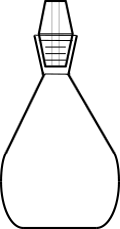Pycnometer

Further information
Density determination of gases with gas ball and piston sampler